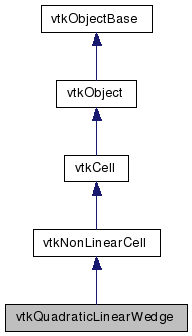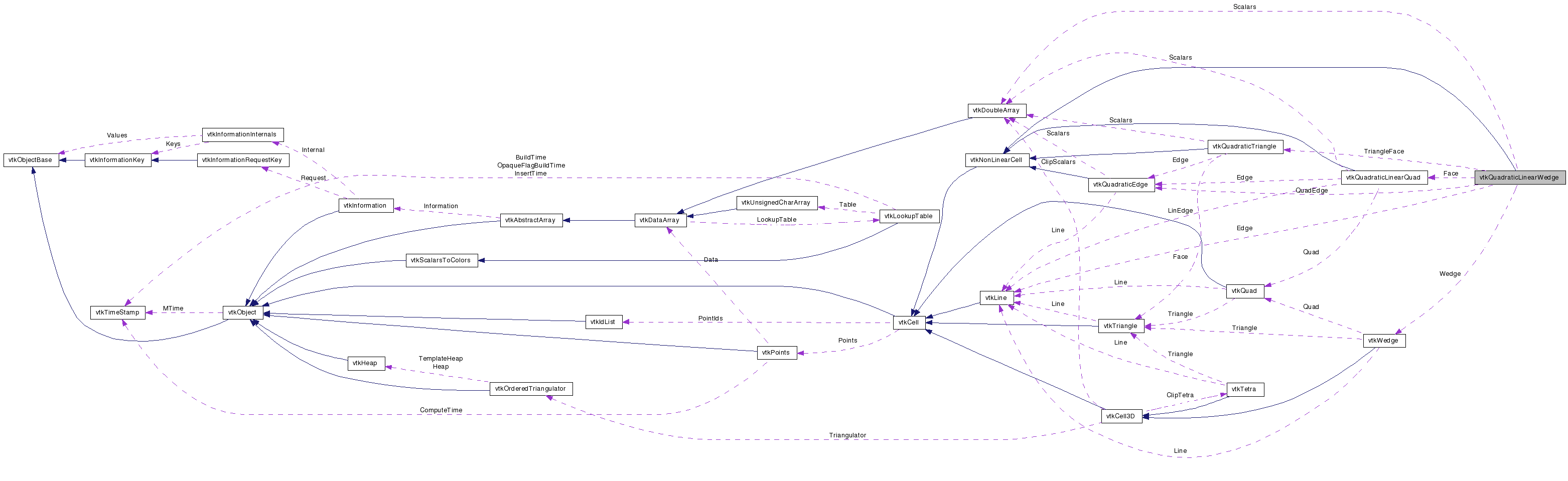# vtkQuadraticLinearWedge Class Reference

`#include <vtkQuadraticLinearWedge.h>`

Inheritance diagram for vtkQuadraticLinearWedge:[legend]
Collaboration diagram for vtkQuadraticLinearWedge:[legend]

## Detailed Description

cell represents a, 12-node isoparametric wedge

vtkQuadraticLinearWedge is a concrete implementation of vtkNonLinearCell to represent a three-dimensional, 12-node isoparametric linear quadratic wedge. The interpolation is the standard finite element, quadratic isoparametric shape function in xy - layer and the linear functions in z - direction. The cell includes mid-edge node in the triangle edges. The ordering of the 12 points defining the cell is point ids (0-5,6-12) where point ids 0-5 are the six corner vertices of the wedge; followed by six midedge nodes (6-12). Note that these midedge nodes correspond lie on the edges defined by (0,1), (1,2), (2,0), (3,4), (4,5), (5,3). The Edges (0,3), (1,4), (2,5) dont have midedge nodes.

Thanks:
Thanks to Soeren Gebbert who developed this class and integrated it into VTK 5.0.
Tests:

Definition at line 53 of file vtkQuadraticLinearWedge.h.

static void InterpolationFunctions (double pcoords, double weights)
static void InterpolationDerivs (double pcoords, double derivs)
static int * GetEdgeArray (int edgeId)
static int * GetFaceArray (int faceId)
virtual void InterpolateFunctions (double pcoords, double weights)
virtual void InterpolateDerivs (double pcoords, double derivs)

## Public Types

typedef vtkNonLinearCell Superclass

## Public Member Functions

virtual const char * GetClassName ()
virtual int IsA (const char *type)
void PrintSelf (ostream &os, vtkIndent indent)
int CellBoundary (int subId, double pcoords, vtkIdList *pts)
int GetParametricCenter (double pcoords)
void JacobianInverse (double pcoords, double **inverse, double derivs)
int GetCellType ()
int GetCellDimension ()
int GetNumberOfEdges ()
int GetNumberOfFaces ()
vtkCellGetEdge (int edgeId)
vtkCellGetFace (int faceId)
void Contour (double value, vtkDataArray *cellScalars, vtkPointLocator *locator, vtkCellArray *verts, vtkCellArray *lines, vtkCellArray *polys, vtkPointData *inPd, vtkPointData *outPd, vtkCellData *inCd, vtkIdType cellId, vtkCellData *outCd)
int EvaluatePosition (double x, double *closestPoint, int &subId, double pcoords, double &dist2, double *weights)
void EvaluateLocation (int &subId, double pcoords, double x, double *weights)
int Triangulate (int index, vtkIdList *ptIds, vtkPoints *pts)
void Derivatives (int subId, double pcoords, double *values, int dim, double *derivs)
virtual double * GetParametricCoords ()
void Clip (double value, vtkDataArray *cellScalars, vtkPointLocator *locator, vtkCellArray *tetras, vtkPointData *inPd, vtkPointData *outPd, vtkCellData *inCd, vtkIdType cellId, vtkCellData *outCd, int insideOut)
int IntersectWithLine (double p1, double p2, double tol, double &t, double x, double pcoords, int &subId)

## Static Public Member Functions

static int IsTypeOf (const char *type)
static vtkQuadraticLinearWedgeSafeDownCast (vtkObject *o)

## Protected Attributes

vtkLineEdge
vtkWedgeWedge
vtkDoubleArrayScalars

## Member Typedef Documentation

Reimplemented from vtkNonLinearCell.

Definition at line 57 of file vtkQuadraticLinearWedge.h.

## Constructor & Destructor Documentation

 vtkQuadraticLinearWedge::vtkQuadraticLinearWedge ( ) ` [protected]`

 vtkQuadraticLinearWedge::~vtkQuadraticLinearWedge ( ) ` [protected]`

## Member Function Documentation

 static vtkQuadraticLinearWedge* vtkQuadraticLinearWedge::New ( ) ` [static]`

Create an object with Debug turned off, modified time initialized to zero, and reference counting on.

Reimplemented from vtkObject.

 virtual const char* vtkQuadraticLinearWedge::GetClassName ( ) ` [virtual]`

Reimplemented from vtkNonLinearCell.

 static int vtkQuadraticLinearWedge::IsTypeOf ( const char * name ) ` [static]`

Return 1 if this class type is the same type of (or a subclass of) the named class. Returns 0 otherwise. This method works in combination with vtkTypeRevisionMacro found in vtkSetGet.h.

Reimplemented from vtkNonLinearCell.

 virtual int vtkQuadraticLinearWedge::IsA ( const char * name ) ` [virtual]`

Return 1 if this class is the same type of (or a subclass of) the named class. Returns 0 otherwise. This method works in combination with vtkTypeRevisionMacro found in vtkSetGet.h.

Reimplemented from vtkNonLinearCell.

 static vtkQuadraticLinearWedge* vtkQuadraticLinearWedge::SafeDownCast ( vtkObject * o ) ` [static]`

Reimplemented from vtkNonLinearCell.

 void vtkQuadraticLinearWedge::PrintSelf ( ostream & os, vtkIndent indent ) ` [virtual]`

Methods invoked by print to print information about the object including superclasses. Typically not called by the user (use Print() instead) but used in the hierarchical print process to combine the output of several classes.

Reimplemented from vtkNonLinearCell.

 int vtkQuadraticLinearWedge::GetCellType ( ) ` [inline, virtual]`

Implement the vtkCell API. See the vtkCell API for descriptions of these methods.

Implements vtkCell.

Definition at line 63 of file vtkQuadraticLinearWedge.h.

 int vtkQuadraticLinearWedge::GetCellDimension ( ) ` [inline, virtual]`

Implement the vtkCell API. See the vtkCell API for descriptions of these methods.

Implements vtkCell.

Definition at line 64 of file vtkQuadraticLinearWedge.h.

 int vtkQuadraticLinearWedge::GetNumberOfEdges ( ) ` [inline, virtual]`

Implement the vtkCell API. See the vtkCell API for descriptions of these methods.

Implements vtkCell.

Definition at line 65 of file vtkQuadraticLinearWedge.h.

 int vtkQuadraticLinearWedge::GetNumberOfFaces ( ) ` [inline, virtual]`

Implement the vtkCell API. See the vtkCell API for descriptions of these methods.

Implements vtkCell.

Definition at line 66 of file vtkQuadraticLinearWedge.h.

 vtkCell* vtkQuadraticLinearWedge::GetEdge ( int edgeId ) ` [virtual]`

Implement the vtkCell API. See the vtkCell API for descriptions of these methods.

Implements vtkCell.

 vtkCell* vtkQuadraticLinearWedge::GetFace ( int faceId ) ` [virtual]`

Implement the vtkCell API. See the vtkCell API for descriptions of these methods.

Implements vtkCell.

 int vtkQuadraticLinearWedge::CellBoundary ( int subId, double pcoords, vtkIdList * pts ) ` [virtual]`

Given parametric coordinates of a point, return the closest cell boundary, and whether the point is inside or outside of the cell. The cell boundary is defined by a list of points (pts) that specify a face (3D cell), edge (2D cell), or vertex (1D cell). If the return value of the method is != 0, then the point is inside the cell.

Implements vtkCell.

 void vtkQuadraticLinearWedge::Contour ( double value, vtkDataArray * cellScalars, vtkPointLocator * locator, vtkCellArray * verts, vtkCellArray * lines, vtkCellArray * polys, vtkPointData * inPd, vtkPointData * outPd, vtkCellData * inCd, vtkIdType cellId, vtkCellData * outCd ) ` [virtual]`

The quadratic linear wege is splitted into 4 linear wedges, each of them is contoured by a provided scalar value

Implements vtkCell.

 int vtkQuadraticLinearWedge::EvaluatePosition ( double x, double * closestPoint, int & subId, double pcoords, double & dist2, double * weights ) ` [virtual]`

The quadratic linear wege is splitted into 4 linear wedges, each of them is contoured by a provided scalar value

Implements vtkCell.

 void vtkQuadraticLinearWedge::EvaluateLocation ( int & subId, double pcoords, double x, double * weights ) ` [virtual]`

The quadratic linear wege is splitted into 4 linear wedges, each of them is contoured by a provided scalar value

Implements vtkCell.

 int vtkQuadraticLinearWedge::Triangulate ( int index, vtkIdList * ptIds, vtkPoints * pts ) ` [virtual]`

The quadratic linear wege is splitted into 4 linear wedges, each of them is contoured by a provided scalar value

Implements vtkCell.

 void vtkQuadraticLinearWedge::Derivatives ( int subId, double pcoords, double * values, int dim, double * derivs ) ` [virtual]`

The quadratic linear wege is splitted into 4 linear wedges, each of them is contoured by a provided scalar value

Implements vtkCell.

 virtual double* vtkQuadraticLinearWedge::GetParametricCoords ( ) ` [virtual]`

The quadratic linear wege is splitted into 4 linear wedges, each of them is contoured by a provided scalar value

Reimplemented from vtkCell.

 void vtkQuadraticLinearWedge::Clip ( double value, vtkDataArray * cellScalars, vtkPointLocator * locator, vtkCellArray * tetras, vtkPointData * inPd, vtkPointData * outPd, vtkCellData * inCd, vtkIdType cellId, vtkCellData * outCd, int insideOut ) ` [virtual]`

Clip this quadratic linear wedge using scalar value provided. Like contouring, except that it cuts the hex to produce linear tetrahedron.

Implements vtkCell.

 int vtkQuadraticLinearWedge::IntersectWithLine ( double p1, double p2, double tol, double & t, double x, double pcoords, int & subId ) ` [virtual]`

Line-edge intersection. Intersection has to occur within [0,1] parametric coordinates and with specified tolerance.

Implements vtkCell.

 int vtkQuadraticLinearWedge::GetParametricCenter ( double pcoords ) ` [inline, virtual]`

Return the center of the quadratic linear wedge in parametric coordinates.

Reimplemented from vtkCell.

Definition at line 157 of file vtkQuadraticLinearWedge.h.

 static void vtkQuadraticLinearWedge::InterpolationFunctions ( double pcoords, double weights ) ` [static]`

 static void vtkQuadraticLinearWedge::InterpolationDerivs ( double pcoords, double derivs ) ` [static]`

 virtual void vtkQuadraticLinearWedge::InterpolateFunctions ( double pcoords, double weights ) ` [inline, virtual]`

Deprecated:
Replaced by vtkQuadraticLinearWedge::InterpolateFunctions as of VTK 5.2

Definition at line 120 of file vtkQuadraticLinearWedge.h.

 virtual void vtkQuadraticLinearWedge::InterpolateDerivs ( double pcoords, double derivs ) ` [inline, virtual]`

Deprecated:
Replaced by vtkQuadraticLinearWedge::InterpolateFunctions as of VTK 5.2

Definition at line 124 of file vtkQuadraticLinearWedge.h.

 static int* vtkQuadraticLinearWedge::GetEdgeArray ( int edgeId ) ` [static]`

 static int* vtkQuadraticLinearWedge::GetFaceArray ( int faceId ) ` [static]`

 void vtkQuadraticLinearWedge::JacobianInverse ( double pcoords, double ** inverse, double derivs )

Given parametric coordinates compute inverse Jacobian transformation matrix. Returns 9 elements of 3x3 inverse Jacobian plus interpolation function derivatives.

## Member Data Documentation

 vtkQuadraticEdge* vtkQuadraticLinearWedge::QuadEdge` [protected]`

Definition at line 144 of file vtkQuadraticLinearWedge.h.

 vtkLine* vtkQuadraticLinearWedge::Edge` [protected]`

Definition at line 145 of file vtkQuadraticLinearWedge.h.

 vtkQuadraticTriangle* vtkQuadraticLinearWedge::TriangleFace` [protected]`

Definition at line 146 of file vtkQuadraticLinearWedge.h.

 vtkQuadraticLinearQuad* vtkQuadraticLinearWedge::Face` [protected]`

Definition at line 147 of file vtkQuadraticLinearWedge.h.

 vtkWedge* vtkQuadraticLinearWedge::Wedge` [protected]`

Definition at line 148 of file vtkQuadraticLinearWedge.h.

 vtkDoubleArray* vtkQuadraticLinearWedge::Scalars` [protected]`

Definition at line 149 of file vtkQuadraticLinearWedge.h.

The documentation for this class was generated from the following file:

Generated on Sat Dec 27 13:45:56 2008 for VTK by1.5.6